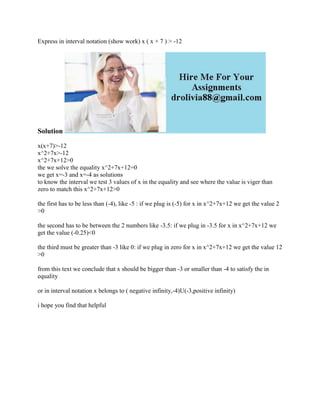Diese Präsentation wurde erfolgreich gemeldet.

# Express in interval notation (show work) x ( x + 7 ) - -12Solution.docx

Anzeige
Anzeige
Anzeige
Anzeige
Anzeige
Anzeige
Anzeige
Anzeige
Anzeige
Anzeige
Anzeige×

1 von 2 Anzeige

# Express in interval notation (show work) x ( x + 7 ) - -12Solution.docx

Express in interval notation (show work) x ( x + 7 ) > -12
Solution
x(x+7)>-12
x^2+7x>-12
x^2+7x+12>0
the we solve the equality x^2+7x+12=0
we get x=-3 and x=-4 as solutions
to know the interval we test 3 values of x in the equality and see where the value is viger than zero to match this x^2+7x+12>0
the first has to be less than (-4), like -5 : if we plug is (-5) for x in x^2+7x+12 we get the value 2 >0
the second has to be between the 2 numbers like -3.5: if we plug in -3.5 for x in x^2+7x+12 we get the value (-0.25)<0
the third must be greater than -3 like 0: if we plug in zero for x in x^2+7x+12 we get the value 12 >0
from this text we conclude that x should be bigger than -3 or smaller than -4 to satisfy the in equality
or in interval notation x belongs to ( negative infinity,-4)U(-3,positive infinity)
i hope you find that helpful
.

Express in interval notation (show work) x ( x + 7 ) > -12
Solution
x(x+7)>-12
x^2+7x>-12
x^2+7x+12>0
the we solve the equality x^2+7x+12=0
we get x=-3 and x=-4 as solutions
to know the interval we test 3 values of x in the equality and see where the value is viger than zero to match this x^2+7x+12>0
the first has to be less than (-4), like -5 : if we plug is (-5) for x in x^2+7x+12 we get the value 2 >0
the second has to be between the 2 numbers like -3.5: if we plug in -3.5 for x in x^2+7x+12 we get the value (-0.25)<0
the third must be greater than -3 like 0: if we plug in zero for x in x^2+7x+12 we get the value 12 >0
from this text we conclude that x should be bigger than -3 or smaller than -4 to satisfy the in equality
or in interval notation x belongs to ( negative infinity,-4)U(-3,positive infinity)
i hope you find that helpful
.

Anzeige
Anzeige

### Express in interval notation (show work) x ( x + 7 ) - -12Solution.docx

1. 1. Express in interval notation (show work) x ( x + 7 ) > -12 Solution x(x+7)>-12 x^2+7x>-12 x^2+7x+12>0 the we solve the equality x^2+7x+12=0 we get x=-3 and x=-4 as solutions to know the interval we test 3 values of x in the equality and see where the value is viger than zero to match this x^2+7x+12>0 the first has to be less than (-4), like -5 : if we plug is (-5) for x in x^2+7x+12 we get the value 2 >0 the second has to be between the 2 numbers like -3.5: if we plug in -3.5 for x in x^2+7x+12 we get the value (-0.25)<0 the third must be greater than -3 like 0: if we plug in zero for x in x^2+7x+12 we get the value 12 >0 from this text we conclude that x should be bigger than -3 or smaller than -4 to satisfy the in equality or in interval notation x belongs to ( negative infinity,-4)U(-3,positive infinity) i hope you find that helpful